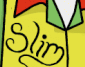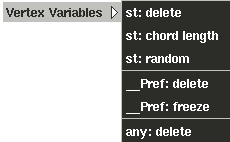##Reference Geometry

### Introduction

In order to solve the problem of surfaces which deform within a rigid projective texture space, we provide the option to output reference geometry. Certain appearances (like magicSurf.slo) are able to use reference geometry to eliminate the texture swimming effect caused by deformations. Shading networks can be built in Slim that take advantage of reference geometry.

### Outputting Reference Geomtery

The main way of dealing with reference geometry in MTOR means setting reference geometry via the vertex variables. MTOR uses reference geometry associated with an object to map textures and procedural shaders onto a deforming object. For accurate results, the coordinate system used for projecting a texture should be parented under the deforming object. (There's a tutorial that covers reference geometry here.)

##### _Pref:freezeThis is the method by which to create reference geometry using Slim shaders. It's quick and easy. Put the deforming model it the starting position, the position that you want the projections to stick at. You may need to slide the time slider to a different frame to accomplish this, but don't worry. "Pref:freeze" operates independently of the time slider. With the object in its starting position, first select the object, and second invoke:
RenderMan-> Vertex Variables-> _Pref:freeze
That's it! Now the object can be animated  and rendered while retaining its reference geometry. The reference geometry won't go away until you select the object and delete the reference geomtery with the "_Pref:delete" command.

A shader must be told to use the reference geometry. Many shaders have parameters that allow a user to turn the use of reference geometry on and off. In Slim manifolds the parameter "IgnorePref" can be set to ignore reference geometry. The default setting uses reference geometry. Other shaders have parameters called "sticky" which do much the same thing.

So, if reference geometry is output and a shader is told to use it, then textures can be projected onto deforming geometry with nice results. The reference geometry approach is somewhat expensive, however, in that it doubles the amount of information that the renderer must manage for every geometric primitive with this feature enabled. You needn't enable Reference Geometry unless you are using one of the non-parametric projections and your object is undergoing deformations. If standard ST surface parameterization or projections are sufficient for your model, use them - they're cheaper.

### Writing shaders which use Reference Geometry

Here's a trivial snippet showing the use of reference geometry in your own shaders. Note the following:
• The reference geometry is accessed through a standard variable named __Pref.
• It's often useful to provide an additional shader parameter which controls whether to use __Pref or some other variable (typically a function of P).

mySurf.sl

``` surface
mySurf (
float Sticky = 0;
varying point __Pref = point "object" 0;
)
{
if( Sticky == 0 )
Ci = color noise( P );
else
Ci = color noise( __Pref );
}
```

mySurf.sli

``` #sli 1.0
desc = "A trivial example for using __Pref";
flags = [UsesPref];
param Sticky = {
desc = "If reference geometry is output into the RIB stream, this controls
whether to pay attention to it or not.";
type = switch;
};
```